TitleCollege Algebra
Equations Involving Rational Exponents

WTAMU > Virtual Math Lab > College AlgebraLearning Objectives

 After completing this tutorial, you should be able to: Solve radical equations. Solve equations that have rational exponents.IntroductionTutorial

Step 1:  Isolate one of the radicals.

 In other words, get one radical on one side and everything else on the other using inverse operations. In some problems there is only one radical.  However, there are some problems that have more than one radical.  In these problems make sure you isolate just one.

 The inverse operation to a radical or a root is to raise it to an exponent.  Which exponent?  Good question, it would be the exponent that matches the index or root number on your radical.  In other words, if you had a square root, you would have to square it to get rid of it.  If you had a cube root, you would have to cube it to get rid of it,  and so forth.  You can raise both sides to the 2nd power, 10th power, hundredth power, etc.  As long as you do the same thing to both sides of the equation, the two sides will remain equal to each other.

Step 3: If you still have a radical sign left, repeat steps 1 and 2.

 Sometimes you start out with two or more radicals in your equation.  If that is the case and you have at least one nonradical term, you will probably have to repeat steps 1 and 2.

Step 4: Solve the remaining equation.

 The equations in this tutorial will lead to either a linear or a quadratic equation. If you need a review on solving linear equations, feel free to go to Tutorial 14: Linear Equations in One Variable.  If you need a review on solving quadratic equations, feel free to go to Tutorial 17:  Quadratic Equations.

Step 5:  Check for extraneous solutions.

 When solving radical equations, extra solutions may come up when you raise both sides to an even power.  These extra solutions are called extraneous solutions. If a value is an extraneous solution, it is not a solution to the original problem. In radical equations, you check for extraneous solutions by plugging in the values you found back into the original problem. If the left side does not equal the right side, then you have an extraneous solution.Example 1: Solve the radical equation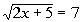.

 Step 1:  Isolate one of the radicals.

 If you square a square root, it will disappear.  This is what we want to do here so that we can get x out from under the square root and continue to solve for it.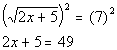*Inverse of taking the sq. root is squaring it

 Step 3: If you still have a radical left, repeat steps 1 and 2.

 No more radicals exist, so we do not have to repeat steps 1 and 2.

 Step 4: Solve the remaining equation.

 In this example, the equation that resulted from squaring both sides turned out to be a linear equation. If you need a review on solving linear equations, feel free to go to Tutorial 14: Linear Equations in One Variable.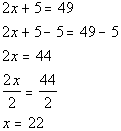*Inverse of add. 5 is sub. 5   *Inverse of mult. by 2 is div. by 2

 Step 5:  Check for extraneous solutions.

 Let's check to see if x = 22 is an extraneous solution: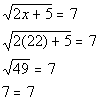*Plugging in 22 for x   *True statement

 Since we got a true statement, x = 22 is not an extraneous solution. There is one solution to this radical equation: x = 22.Example 2: Solve the radical equation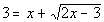.

 Step 1:  Isolate one of the radicals.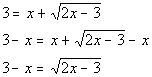*Inverse of add. x is sub. x *Square root is by itself on one side of eq.

 If you square a square root, it will disappear.  This is what we want to do here so that we can get x out from under the square root and continue to solve for it.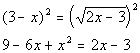*Inverse of taking the sq. root is squaring it *Left side is a binomial squared

 Be careful on this one.  It is VERY TEMPTING to square the left side term by term and get  9 - x squared or 9 + x squared.  However, you need to square it as a side as shown above.  Recall that when you square a binomial you get the first term squared minus twice the product of the two terms plus the second term squared.  If you need a review on squaring a binomial, feel free to go to Tutorial 6: Polynomials.

 Step 3: If you still have a radical left, repeat steps 1 and 2.

 No more radicals exist, so we do not have to repeat steps 1 and 2.

 Step 4: Solve the remaining equation.

 In this example, the equation that resulted from squaring both sides turned out to be a quadratic equation. If you need a review on solving quadratic equations, feel free to go to Tutorial 17:  Quadratic Equations.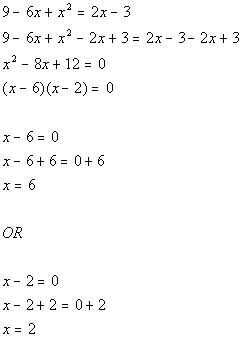*Quad. eq. in standard form *Factor the trinomial *Use Zero-Product Principle *Set 1st factor = 0 and solve             *Set 2nd factor = 0 and solve

 Step 5:  Check for extraneous solutions.

 Let's check to see if x = 6 is an extraneous solution: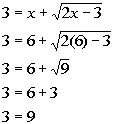*Plugging in 6 for x     *False statement

 Since we got a false statement, x = 6 is an extraneous solution.

 Lets check to see if x = 2 is an extraneous solution: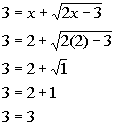*Plugging in 2 for x     *True statement

 Since we got a true statement, x = 2 is a solution. There is only one solution to this radical equation: x = 2.Example 3: Solve the radical equation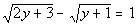.

 Step 1:  Isolate one of the radicals.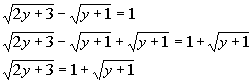*Inverse of sub. sq. root  is add. sq. root *One square root is by itself on one side of eq.

 If you square a square root, it will disappear.  This is what we want to do here so that we can get y out from under the square root and continue to solve for it.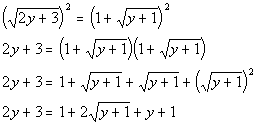*Inverse of taking the sq. root is squaring it *Right side is a binomial squared

 Be careful on this one.  It is VERY TEMPTING to square the right side term by term and get  1 + (y + 1).  However, you need to square it as a side as shown above.  Recall that when you square a binomial you get the first term squared plus twice the product of the two terms plus the second term squared.  If you need a review on squaring a binomial, feel free to go to Tutorial 6: Polynomials.

 Step 3: If you still have a radical left, repeat steps 1 and 2.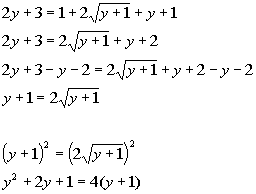*Inverse of add. y and 2 is sub. y and 2 *Square root is by itself on one side of eq.   *Inverse of taking the sq. root is squaring it *Left side is a binomial squared

 Be careful on this one.  It is VERY TEMPTING to square the left side term by term and get  y squared plus 1.  However, you need to square it as a side as shown above.  Recall that when you square a binomial you get the first term squared plus twice the product of the two terms plus the second term squared.  If you need a review on squaring a binomial, feel free to go to Tutorial 6: Polynomials.

 Step 4: Solve the remaining equation.

 In this example, the equation that resulted from squaring both sides turned out to be a quadratic equation. If you need a review on solving quadratic equations, feel free to go to Tutorial 17:  Quadratic Equations.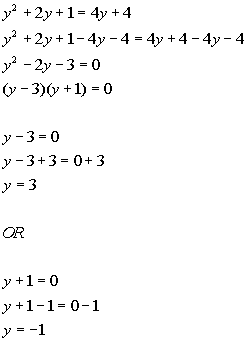*Quad. eq. in standard form *Factor the trinomial *Use Zero-Product Principle *Set 1st factor = 0 and solve           *Set 2nd factor = 0 and solve

 Step 5:  Check for extraneous solutions.

 Let's check to see if y = 3 is an extraneous solution: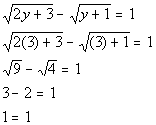*Plugging in 3 for y     *True statement

 Since we got a true statement, y = 3 is a solution.

 Let's check to see if y = -1 is an extraneous solution: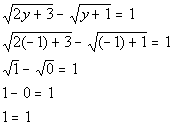*Plugging in -1 for y     *True statement

 Since we got a true statement, y = -1 is a solution. There are two solutions to this radical equation: y = 3 and y = -1.

 Solving Equations that have a Rational Exponent  AND can be written in the form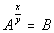where B is any real number.

Step 1:  Isolate the base with the rational exponent.

 In other words get the base with the rational exponent on one side and everything else on the other using inverse operations.

Step 2: Get rid of the rational exponent.

 The inverse operation to a rational exponent is to raise it to the reciprocal of that exponent. This will get rid of the rational exponent on the expression.  For example, if the rational exponent is 2/3, then the inverse operation is to raise both sides to the 3/2 power. You can raise both sides to ANY power.  As long as you do the same thing to both sides of the equation, the two sides will remain equal to each other.

Step 3: Solve the remaining equation.

 The equations in this tutorial will lead to either a linear or a quadratic equation. If you need a review on solving linear equations, feel free to go to Tutorial 14: Linear Equations in One Variable.  If you need a review on solving quadratic equations, feel free to go to Tutorial 17:  Quadratic Equations.

Step 4:  Check for extraneous solutions.

 When solving equations with rational exponents, extra solutions may come up when you raise both sides to an even power.  These extra solutions are called extraneous solutions.  If a value is an extraneous solution, it is not a solution to the original problem. In equations with rational exponents you check for extraneous solutions by plugging in the values you found back into the original problem. If the left side does not equal the right side than you have an extraneous solution.Example 4: Solve the rational exponent  equation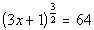.

 The base with the rational exponent  is already isolated.

 If you raise an expression that has a rational exponent to the reciprocal of that rational exponent, the exponent will disappear.   This is what we want to do here so that we can get x out from under the rational exponent and continue to solve for it.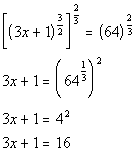*Inverse of taking it to the  3/2 power is taking it to the 2/3 power

 Step 3: Solve the remaining equation.

 In this example the equation that resulted from raising both sides to the 2/3 power turned out to be a linear equation. If you need a review on solving linear equations, feel free to go to Tutorial 14: Linear Equations in One Variable.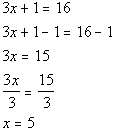*Inverse of add. 1 is sub. 1   *Inverse of mult. by 3 is div. by 3

 Step 4:  Check for extraneous solutions.

 Let's check to see if x = 5 is an extraneous solution: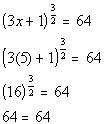*Plugging in 5 for x     *True statement

 Since we got a true statement, x = 5 is a solution. There is one solution to this rational exponent equation: x = 5.Example 5: Solve the rational exponent equation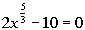.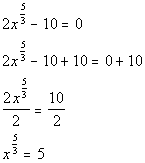*Inverse of sub. 10 is add. 10   *Inverse of mult. by 2 is div. by 2 *rat. exp. expression is by itself on one side of eq.

 If you raise an expression that has a rational exponent to the reciprocal of that rational exponent, the exponent will disappear.   This is what we want to do here so that we can get x out from under the rational exponent and continue to solve for it.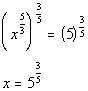*Inverse of taking it to the  5/3 power is taking it to the 3/5 power

 Step 3: Solve the remaining equation.

 In this example, the equation that resulted from raising both sides to the 3/5 power turned out to be a linear equation. Also note that it is already solved for x.  So, we do not have to do anything on this step for this example.

 Step 4:  Check for extraneous solutions.

 Let's check to see if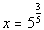is an extraneous solution: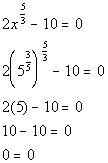*Plugging in 5 to the 3/5 power for x     *True statement

 Since we got a true statement,is a solution. There is one solution to this rational exponent equation:.Example 6: Solve the rational exponent equation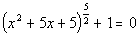.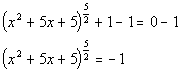*Inverse of add. 1 is sub. 1 *rat. exp. expression is by itself on one side of eq.

 If you raise an expression that has a rational exponent to the reciprocal of that rational exponent, the exponent will disappear.   This is what we want to do here so that we can get x out from under the rational exponent and continue to solve for it.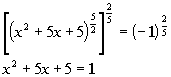*Inverse of taking it to the  5/2 power is taking it to the 2/5 power *The 5th root of -1 is -1 and -1 squared is 1.

 Step 3: Solve the remaining equation.

 In this example, the equation that resulted from squaring both sides turned out to be a quadratic equation. If you need a review on solving quadratic equations, feel free to go to Tutorial 17:  Quadratic Equations.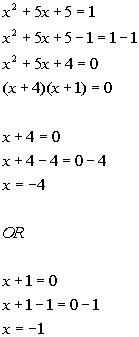*Quad. eq. in standard form *Factor the trinomial *Use Zero-Product Principle *Set 1st factor = 0 and solve           *Set 2nd factor = 0 and solve

 Step 4:  Check for extraneous solutions.

 Lets check to see if x = -4 is an extraneous solution: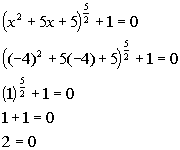*Plugging in -4 for x       *False statement

 Since we got a false statement, x = -4 is an extraneous solution.

 Let's check to see if x = -1 is an extraneous solution: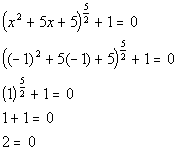*Plugging in -1 for x       *False statement

 Since we got a false statement, x = -1 is an extraneous solution. There is no solution to this rational exponent equation.Practice Problems

 These are practice problems to help bring you to the next level.  It will allow you to check and see if you have an understanding of these types of problems. Math works just like anything else, if you want to get good at it, then you need to practice it.  Even the best athletes and musicians had help along the way and lots of practice, practice, practice, to get good at their sport or instrument.  In fact there is no such thing as too much practice. To get the most out of these, you should work the problem out on your own and then check your answer by clicking on the link for the answer/discussion for that  problem.  At the link you will find the answer as well as any steps that went into finding that answer.Practice Problems 1a - 1b: Solve each radical equation.

 1a.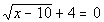(answer/discussion to 1a) 1b.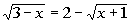(answer/discussion to 1b)Practice Problems 2a - 2b: Solve each rational exponent equation.

 2a.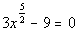(answer/discussion to 2a) 2b.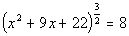(answer/discussion to 2b)Need Extra Help on these Topics?

The following is a webpage that can assist you in the topics that were covered on this page:

 http://www.sosmath.com/algebra/solve/solve0/solve0.html#radical Problems 1,2, 3, & 4 of this part of the webpage helps you with solving equations with radicals.  ONLY do problems 1, 2, 3, & 4.

Go to Get Help Outside the Classroom found in Tutorial 1: How to Succeed in a Math Class for some more suggestions.

WTAMU > Virtual Math Lab > College Algebra

Videos at this site were created and produced by Kim Seward and Virginia Williams Trice.
Last revised on Dec. 16, 2009 by Kim Seward.